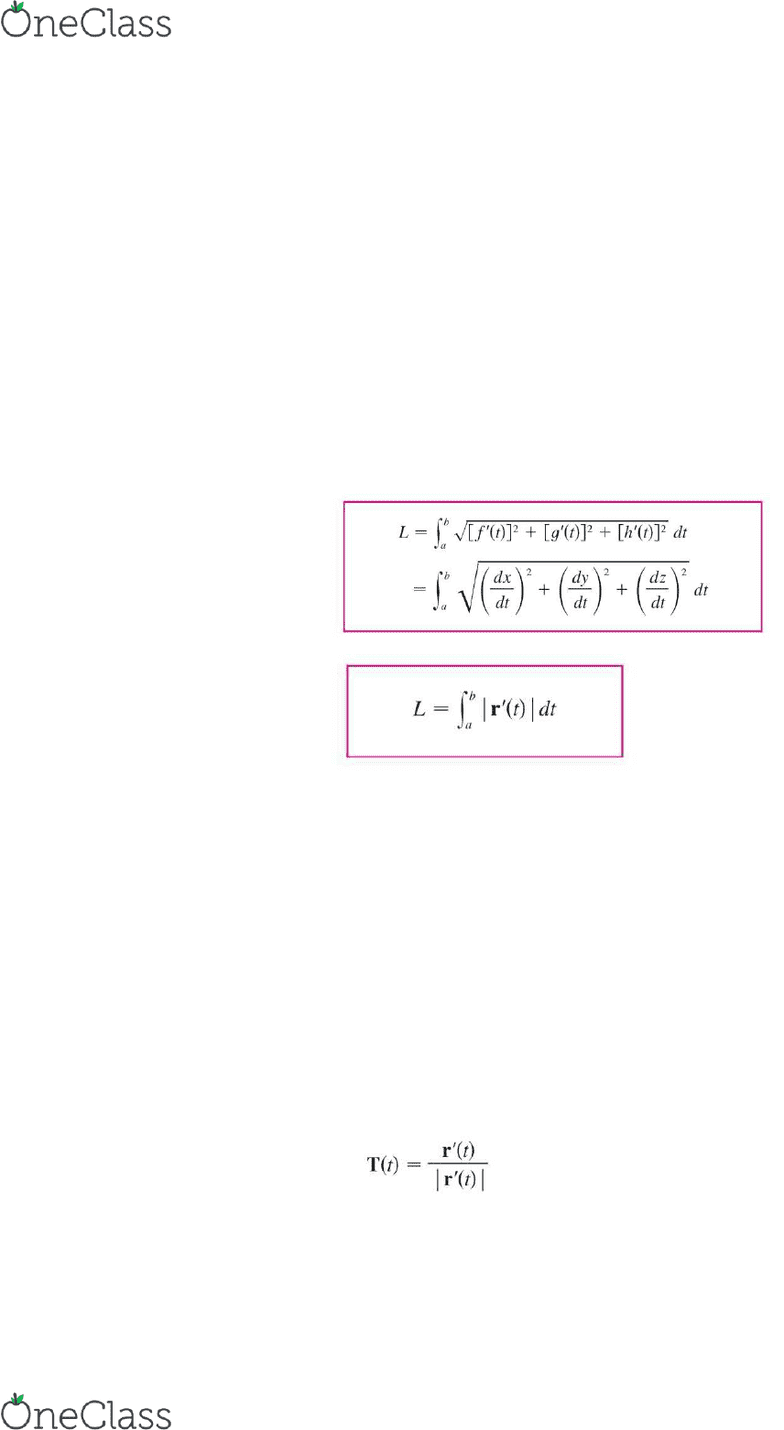Textbook Notes (270,000)
US (100,000)
MATH (40)
Chapter 10.3

MATH 2400 Chapter Notes - Chapter 10.3: Osculating Plane, Osculating Circle, Plane Curve

Department
Mathematics
Course Code
MATH 2400
Professor
Markus Steindl
Chapter
10.3

This preview shows half of the first page. to view the full 3 pages of the document.Anna Pyle
MATH2400 Calculus 3
Textbook: CALCULUS concepts & contexts 4 James Stewart
Chapter 10: Arc Length and Curvature
Arc Length
oThe length of a space curve is defined below:
oIt is useful to parametrize curve with respect to arc length because arc length arises naturally
from the shape of the curve and does not depend on a particular coordinate system
Curvature
oA parametrization r(t) is called smooth on an interval I if r’ is continuous and r’(t) is not equal to
0 on I. It is also called smooth if it has a smooth parametrization. This means it has no sharp
corners or cusps.
oIf C is a smooth curve defined by the vector function r, recall that the unity tangent vector T(t) is
given by the equation shown below and represents the direction of the curve
find more resources at oneclass.com
find more resources at oneclass.com